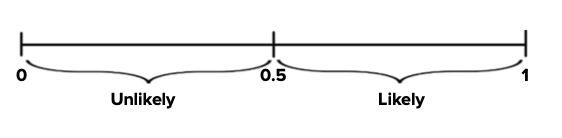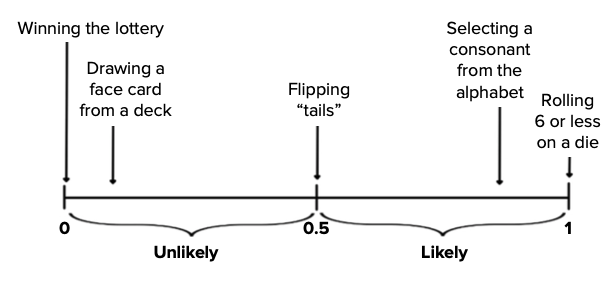+
Common Core: S.CP.1

# Outcomes and Events

Author: Sophia Tutorial
##### Description:

Identify possible outcomes for a given situation.

(more)

Sophia’s self-paced online courses are a great way to save time and money as you earn credits eligible for transfer to many different colleges and universities.*

No credit card required

37 Sophia partners guarantee credit transfer.

299 Institutions have accepted or given pre-approval for credit transfer.

* The American Council on Education's College Credit Recommendation Service (ACE Credit®) has evaluated and recommended college credit for 32 of Sophia’s online courses. Many different colleges and universities consider ACE CREDIT recommendations in determining the applicability to their course and degree programs.

Tutorial
what's covered
This tutorial will cover outcomes and events. Our discussion breaks down as follows:

1. The Difference Between Outcomes and Events
2. Probability Theory

## 1. The Difference Between Outcomes and Events

In probability, we define an outcome as the singular result of some random process.

EXAMPLE

When you roll a die, the outcome is the role of a 1 or a 6, etc. There is only one, singular result.

Outcomes will be different based on the process:

Random Process Outcome
Die rolling Rolling a 5
Flipping a coin Tails
Spinning the wheel on Wheel of Fortune “Bankrupt” (one of the sectors on the wheel)

There's a difference between an outcome and an event. While outcomes only have one result, events can have multiple results.

Random Process Event Outcome
Die rolling Rolling an even number Rolling a 2, 4, or 6
Flipping a coin Tails Tails
Spinning the wheel on Wheel of Fortune Sector over \$500 “\$1,500”

When rolling a die, while an outcome would be a 5, an event could be rolling an even number. That consists of the outcomes 2, 4, and 6.

It could be that an event has one outcome in it, like with the coin flipping: an outcome would be tails, but maybe tails is also considered an event. This is an event with one outcome in it.

On the Wheel of Fortune example, an event such as a sector over \$500, there are several outcomes possible.

terms to know

Outcome
The singular result of a chance experiment.
Event
An outcome or set of outcomes.

## 2. Probability Theory

When you do the random process once, like spinning a wheel, rolling a die, or flipping a coin, that's called a trial.

Probability is going to be defined as the likelihood of a particular event occurring when you do one version of a random process. It is expressed as a number between 0 and 1. Events with probabilities closer to 1 are more likely to occur than events with probabilities closer to 0.

A graph of probability might look like this:The number in the center, which is 0.5, is considered neither likely nor unlikely. It's equally likely to occur as not occur.

brainstorm
What are some events that would fall on this spectrum, from unlikely to likely? Some possible examples might be:• Winning the lottery has a very low probability--almost zero. A zero probability indicates that the event is impossible. It could not happen. Winning the lottery is almost impossible, so it is at the farthest left here.
• Drawing a face card from a deck of cards is further to the left because it is less likely. There are fewer face cards than non-face cards, which means that it would be unlikely to pick a face card.
• Flipping tails on the coin is in the center. There are just as many ways to get tails as not get tails, and so we're going to say that flipping coins numerically has a probability of ½, or 0.5.
• Selecting a letter at random from the alphabet, on the other hand, is quite likely. It's even more likely that you would select a consonant than a vowel, so that would be plotted further to the right.
• To have the highest probability, 1, you need an event that is certain to happen. Rolling 6 or less on a die has to happen--the die result has to be the number 6 or less than that. Therefore, this goes on the farthest right.
terms to know

Trial
Running a chance experiment once (e.g., rolling a die, spinning a spinner, flipping a coin)
Probability
A number between 0 and 1 that denotes the likelihood of an event. Events with probabilities closer to 1 are more likely to occur than events with probabilities closer to 0.

summary
Probability theory discusses the ways to quantify likelihood. Probability is a way to determine how likely certain outcomes or events are when you run a trial of a chance experiment. The difference between outcomes and events is that outcome is the singular result of one trial, whereas an event could be many outcomes, or results, of a single trial.

Good luck!

Source: Adapted from Sophia tutorial by Jonathan Osters.

Terms to Know
Event

An outcome or set of outcomes.

Outcome

The singular result of a chance experiment.

Probability

A number between 0 and 1 that denotes the likelihood of an event. Events with probabilities closer to 1 are more likely to occur than events with probabilities closer to 0.

Trial

Running a chance experiment once (e.g. rolling a die, spinning a spinner, flipping a coin)

Rating